## Question 2 (10%) An electromagnet of flux equal to 5Wb increases uniformly to 20Wb in a period of 2s Calculate the Voltage induc

Question

Question 2 (10%)
An electromagnet of flux equal to 5Wb increases uniformly to 20Wb in a period of 2s Calculate the
Voltage induced in a coil having(6 turys and is stationary in the magnetic field.
(10%)

in progress 0
1 month 2021-08-07T19:58:23+00:00 1 Answers 3 views 0

45 V

Explanation:

Electromagnetic induction occurs when there an electromotive force is induced in a coil due to a change in magnetic flux through the coil.

Mathematically, the induced voltage is given by Faraday-Newmann-Lenz law: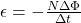where

N is the number of turns in the coil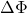is the change in magnetic flux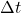is the time elapsed

In this problem,

N = 6 is the number of turns in the coil

The change in magnetic flux is: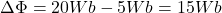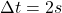is the time elapsed

Substituting, we find the induced voltage: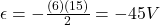And the negative sign only indicates the direction.